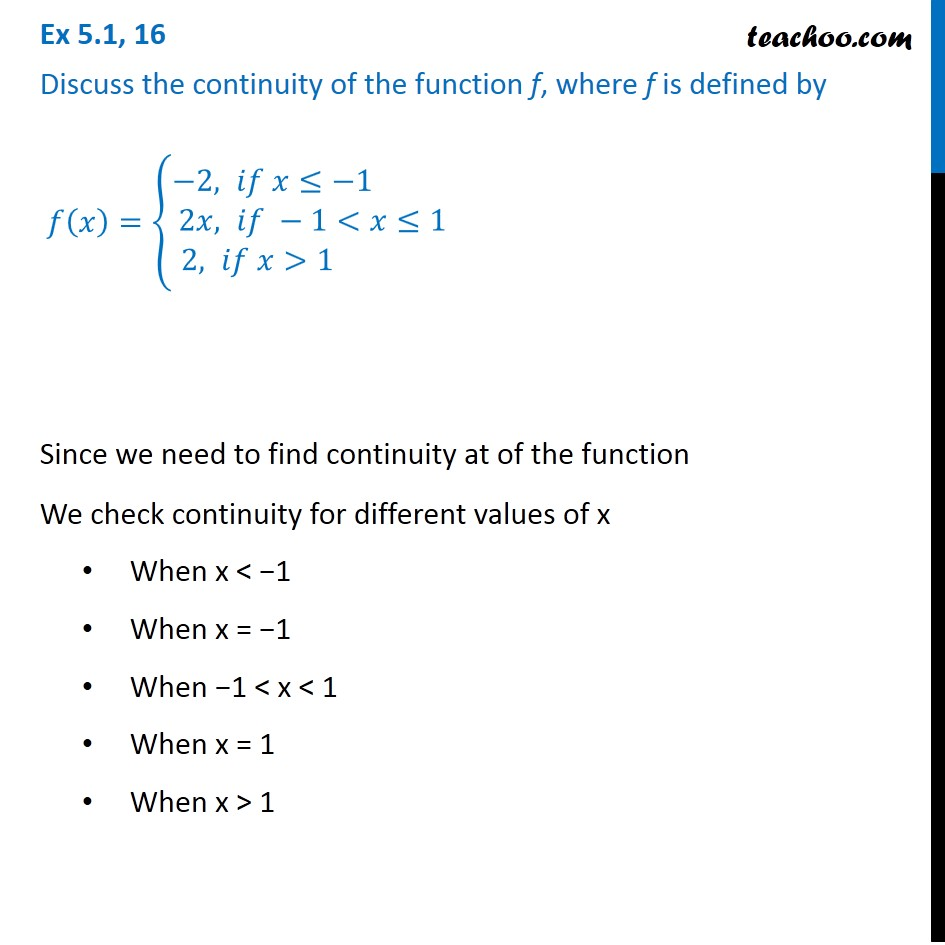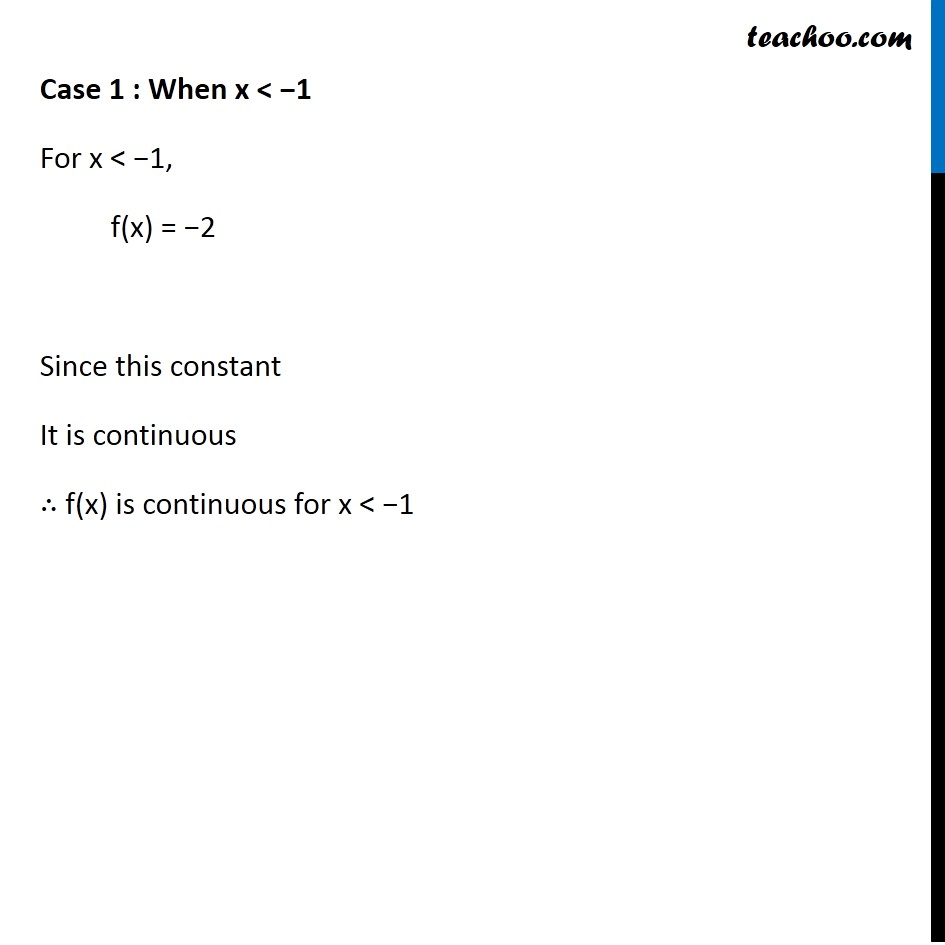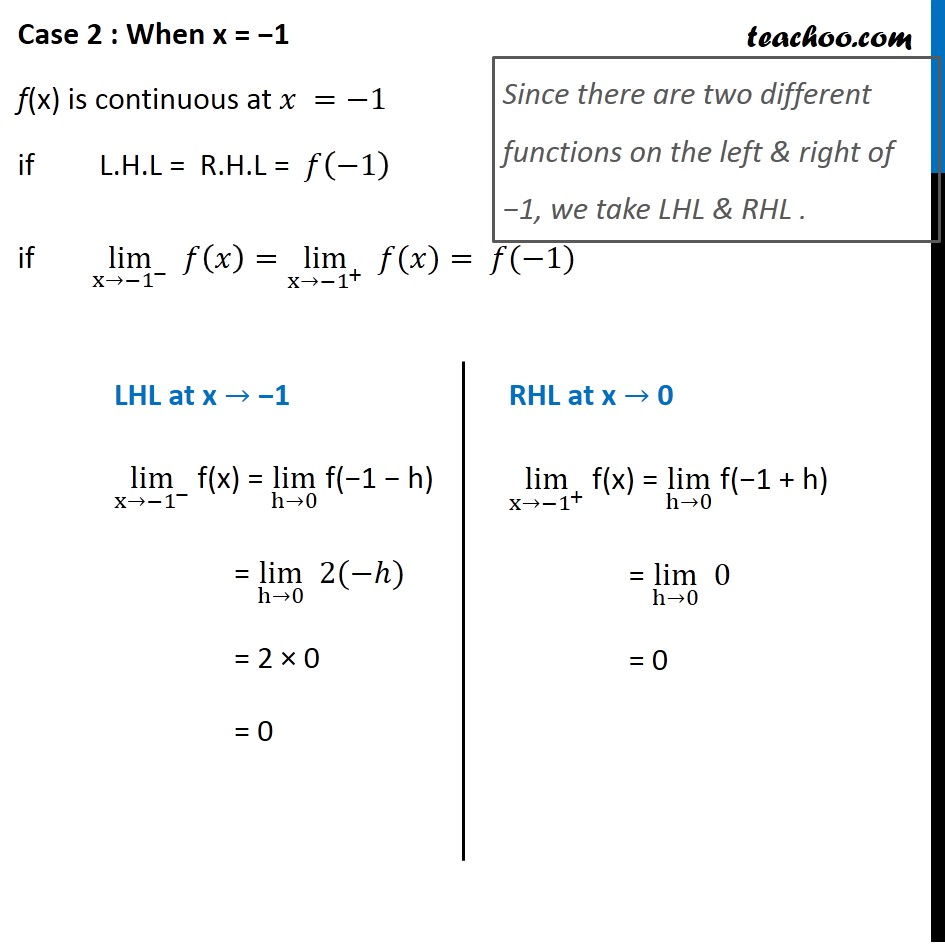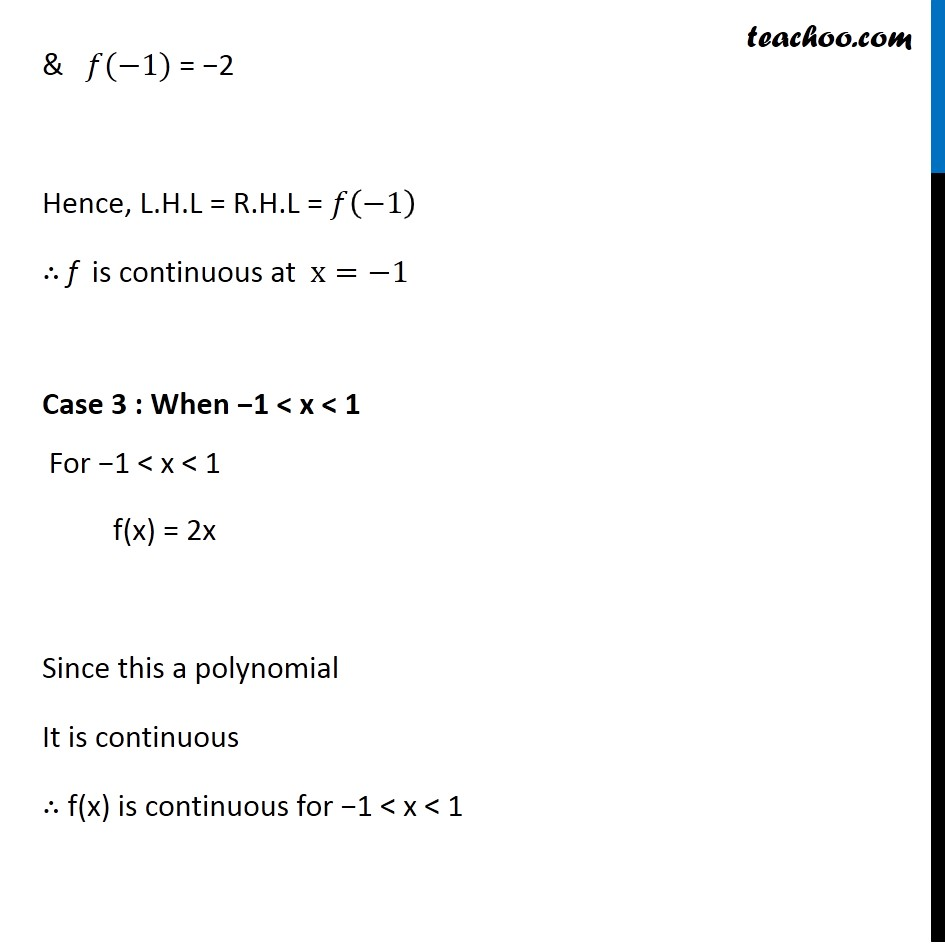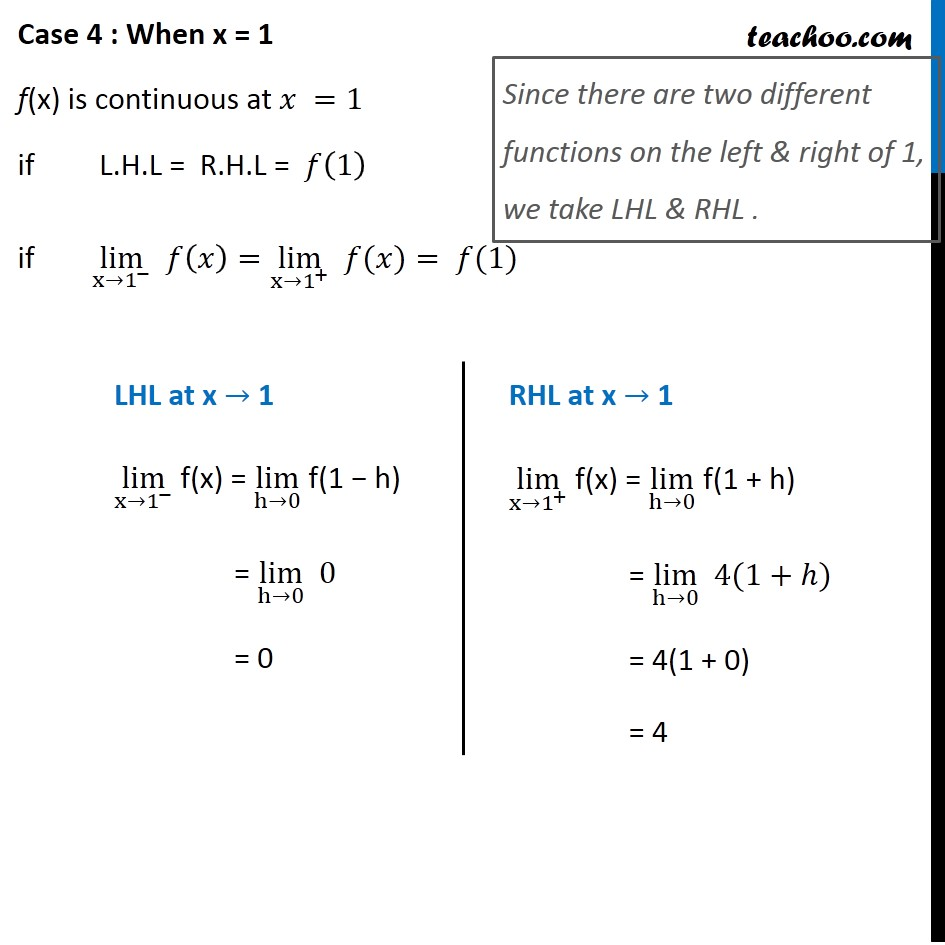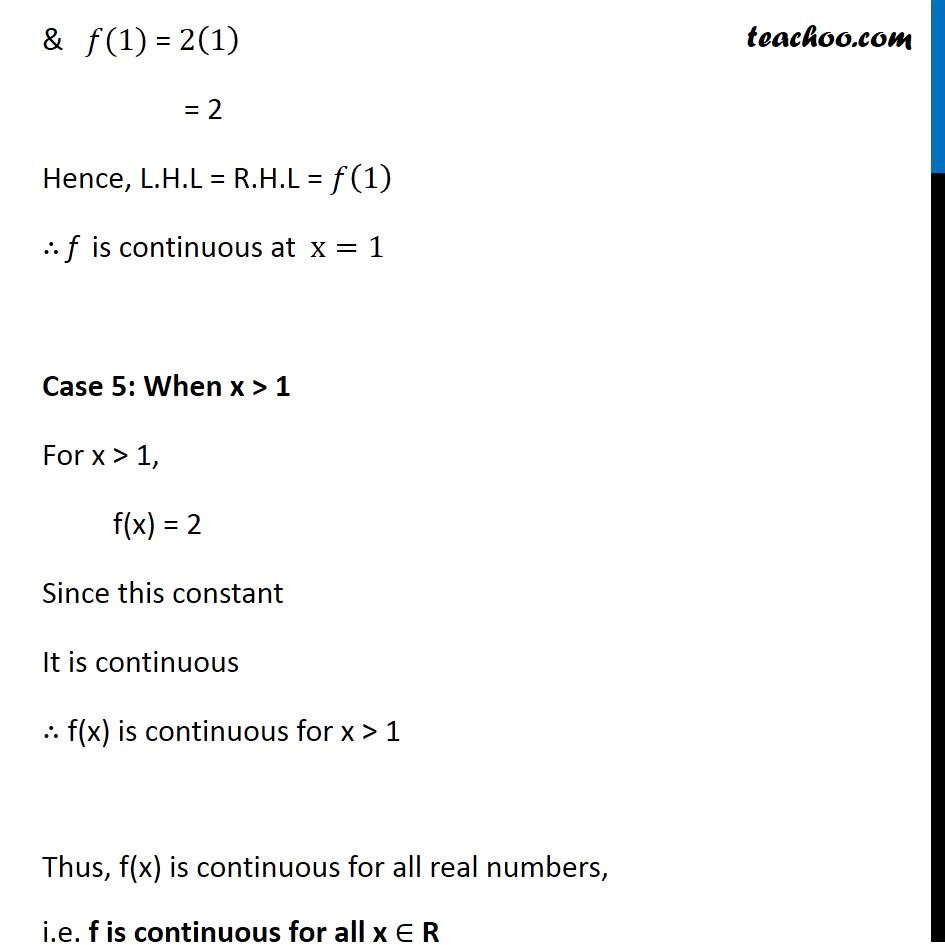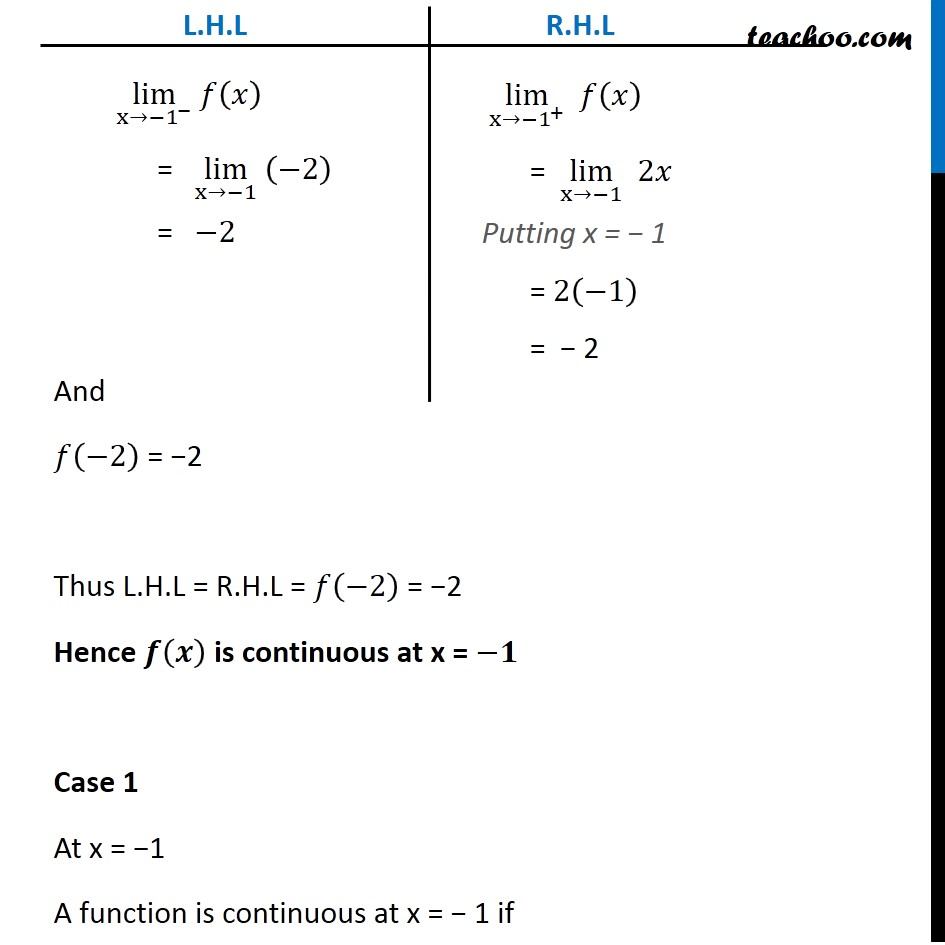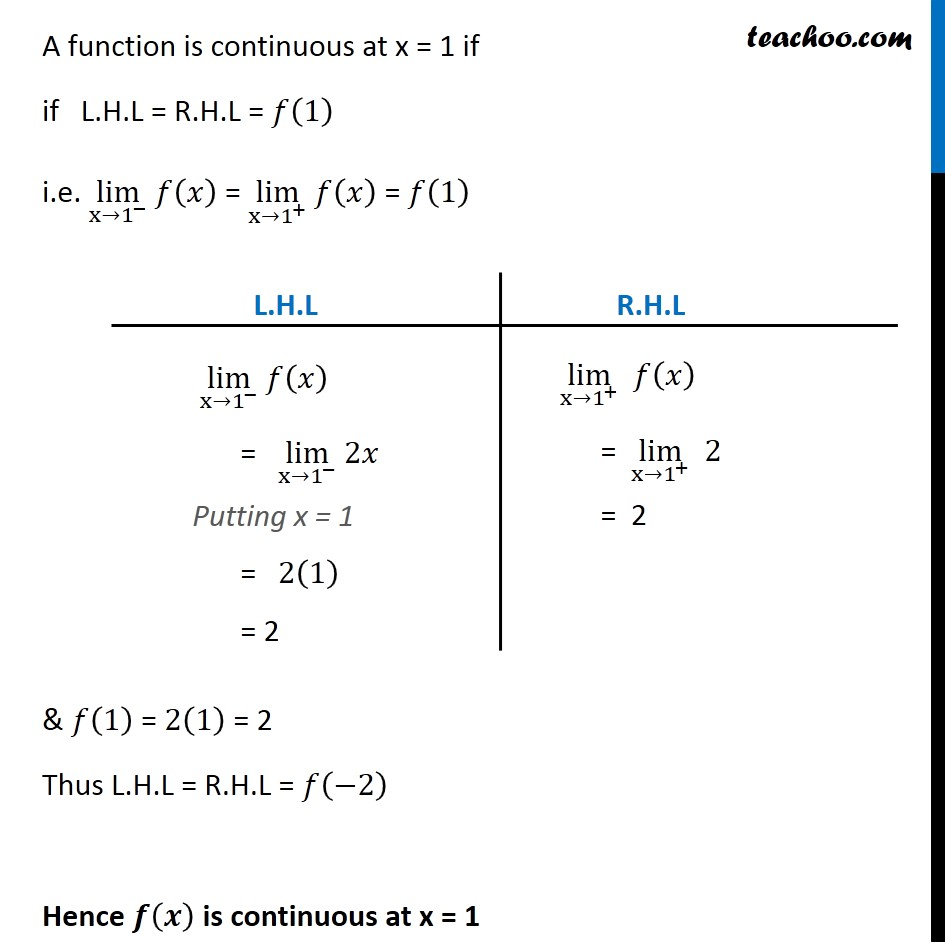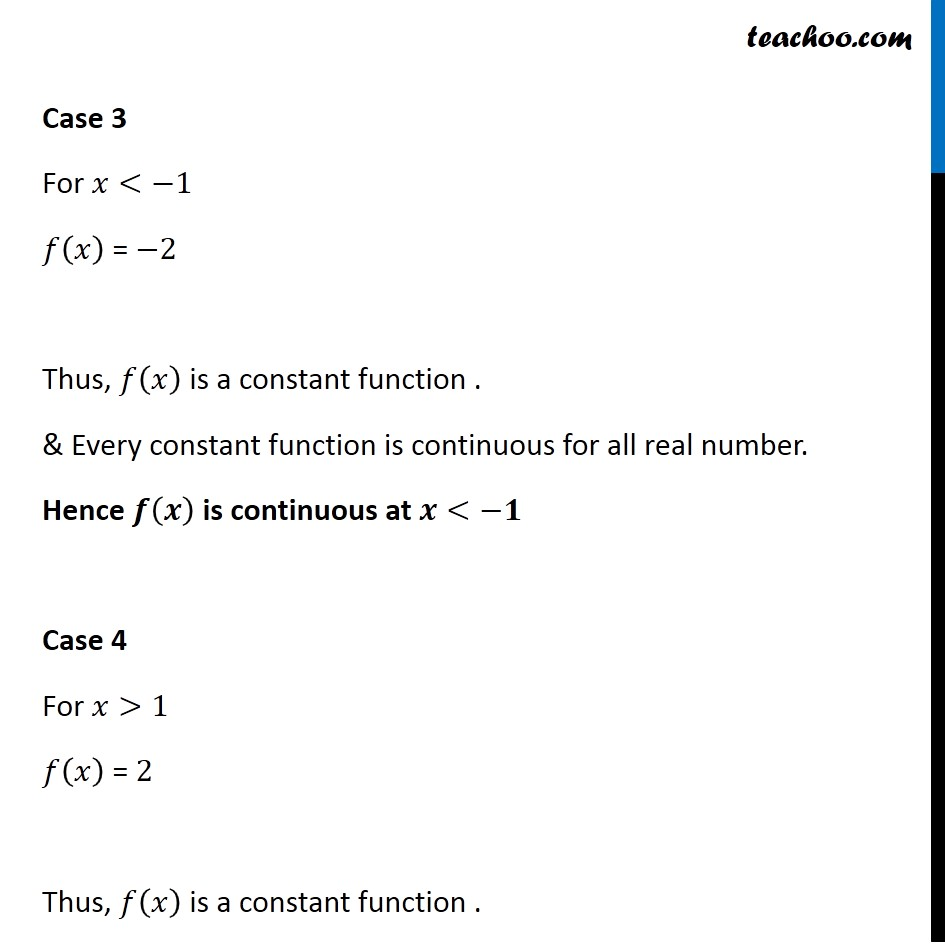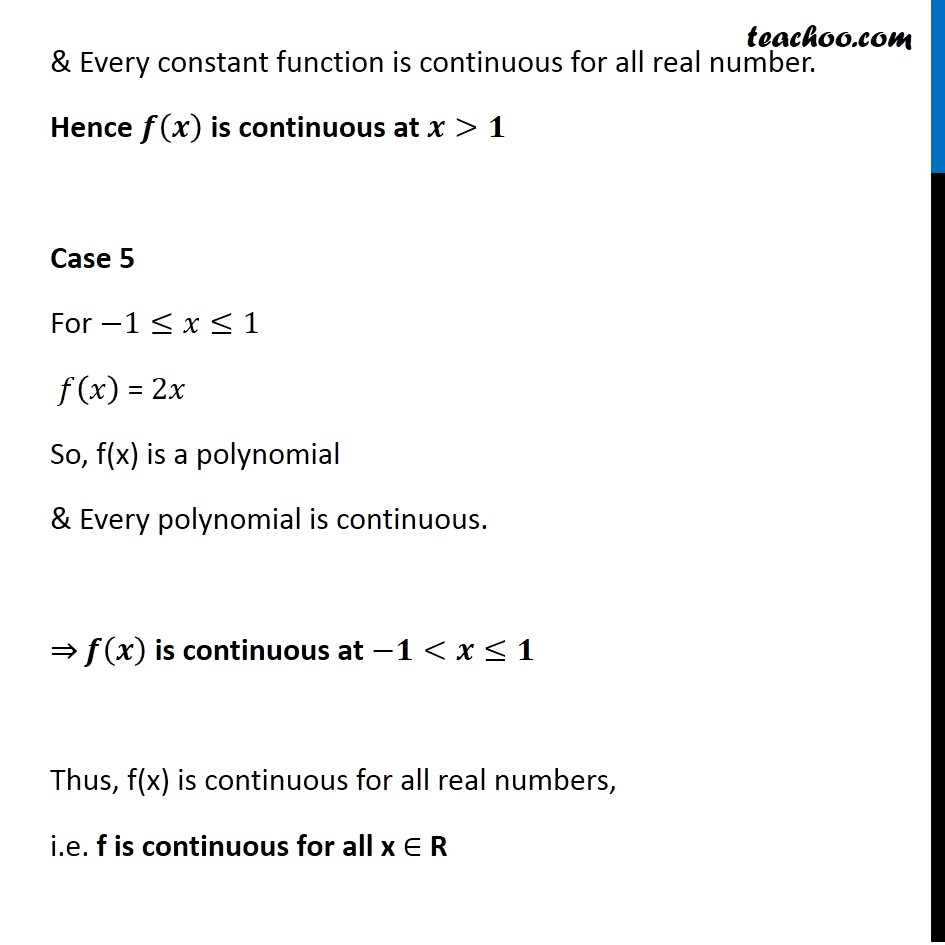1. Chapter 5 Class 12 Continuity and Differentiability (Term 1)
2. Serial order wise
3. Ex 5.1

Transcript

Ex 5.1, 16 Discuss the continuity of the function f, where f is defined by 𝑓(𝑥)={█(−&2, 𝑖𝑓 𝑥≤−1@2&𝑥, 𝑖𝑓 −1<𝑥≤1@2, 𝑖𝑓 𝑥>1 )┤ Since we need to find continuity at of the function We check continuity for different values of x When x < −1 When x = −1 When −1 < x < 1 When x = 1 When x > 1 Case 1 : When x < −1 For x < −1, f(x) = −2 Since this constant It is continuous ∴ f(x) is continuous for x < −1 Case 2 : When x = −1 f(x) is continuous at 𝑥 =−1 if L.H.L = R.H.L = 𝑓(−1) if lim┬(x→〖−1〗^− ) 𝑓(𝑥)=lim┬(x→〖−1〗^+ ) " " 𝑓(𝑥)= 𝑓(−1) Since there are two different functions on the left & right of −1, we take LHL & RHL . LHL at x → −1 lim┬(x→〖−1〗^− ) f(x) = lim┬(h→0) f(−1 − h) = lim┬(h→0) −2 = −2 RHL at x → 0 lim┬(x→〖−1〗^+ ) f(x) = lim┬(h→0) f(−1 + h) = lim┬(h→0) 2(−1+ℎ) = 2(−1+0) = −2 & 𝑓(−1) = −2 Hence, L.H.L = R.H.L = 𝑓(−1) ∴ f is continuous at x = −1 Case 3 : When −1 < x < 1 For −1 < x < 1 f(x) = 2x Since this a polynomial It is continuous ∴ f(x) is continuous for −1 < x < 1 Case 4 : When x = 1 f(x) is continuous at 𝑥 =1 if L.H.L = R.H.L = 𝑓(1) if lim┬(x→1^− ) 𝑓(𝑥)=lim┬(x→1^+ ) " " 𝑓(𝑥)= 𝑓(1) & 𝑓(1) = 2(1) = 2 Since there are two different functions on the left & right of 1, we take LHL & RHL . LHL at x → 1 lim┬(x→1^− ) f(x) = lim┬(h→0) f(1 − h) = lim┬(h→0) 2(1−ℎ) = 2(1−0) = 2 RHL at x → 1 lim┬(x→1^+ ) f(x) = lim┬(h→0) f(1 + h) = lim┬(h→0) 2 = 2 Hence, L.H.L = R.H.L = 𝑓(1) ∴ f is continuous at x=1 Case 5: When x > 1 For x > 1, f(x) = 2 Since this constant It is continuous ∴ f(x) is continuous for x > 1 Thus, f(x) is continuous for all real numbers, i.e. f is continuous for all x ∈ R